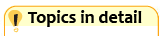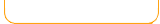SubtractionPictures help you subtract. Number lines help you subtract. Select the fruit that solves the subtraction problem. Subtract with multiple choice fun. Bubble Fun Math Basics Bubble Fun Math Practice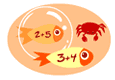Put bubbles around the sea creatures that solves the subtraction problem. (Number ranges)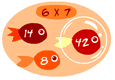Put bubbles around the sea creatures that solves the subtraction problem. (Practice individual numbers) Math Man - Subtract Arcade math game - subtract correctly in this pac-man like game. Racing game - drive into the correct answers.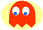1 to 101 to 501 to 100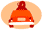11 - 1516 - 20 Match the correct answers. Subtraction arcade game. Match tiles with subtraction and solve the puzzle. Destroy balls that form pairs that subtract to the given number. Catch the stars with your bucket. The stars must subtract to the given number.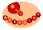to 2 Fruit Splat Subtract Skill BuildersSelect the fruit that solves the subtraction problem. Subtract by numbers.Easy Subtraction arcade game.Advanced

 Learn math the fun way with our free educational games! With animation, music, and lots of fun- kids can enjoy practicing math and increase their math skills and math memory. Topics include: addition, subtraction, multiplication, division, fractions, decimals, numbers, geometry, shapes, early math, integers, time, measurement and much more! Game types involve puzzles, action games, arcade style, driving games, swimming, matching, memory and many more varieties for all kinds of learners and abilities. You can also find math videos and math game demonstrations at our math channel on youtube: